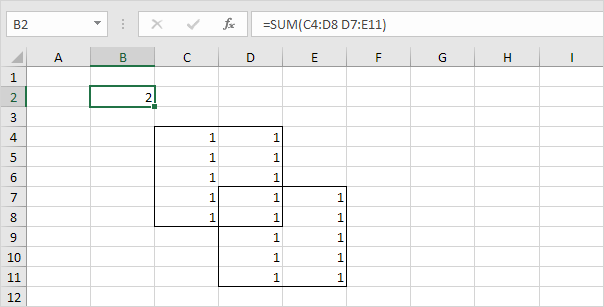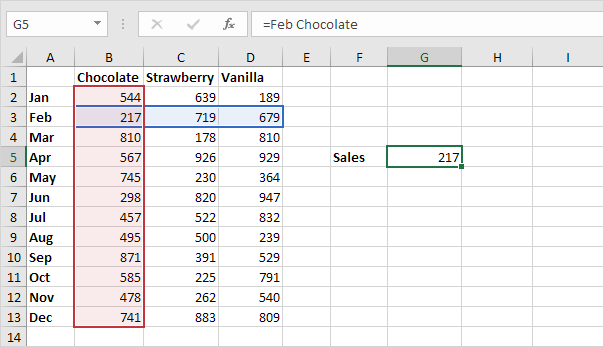# Union and Intersect

This example illustrates how to use the union and intersect operator (borders below for illustration only) in Excel.

1. The union operator (comma) adds two ranges.Explanation: the SUM function reduces to =SUM(C4:D8) + SUM(D7:E11), 20.

2. The intersect operator (single space) returns the intersection of two ranges.Explanation: the SUM function reduces to =SUM(D7:D8), 2.

Let’s look at a few cool examples.

3. Excel automatically adds union operators (commas). For example, type =SUM( and hold down CTRL to select the ranges below.Note: press Enter to insert this function. Excel adds the closing parentheses for you.

4. You can use the intersect operator (space) to return the intersection of two named ranges.Note: the named range Feb refers to the range B3:D3 and the named range Chocolate refers to the range B2:B13.